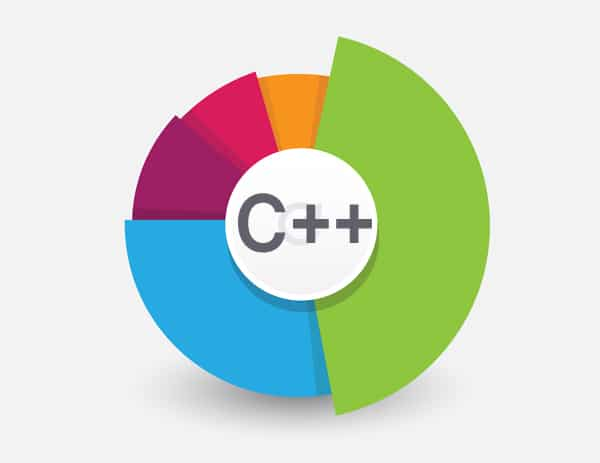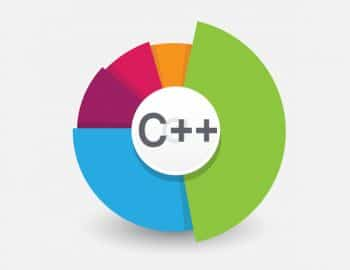# Understanding Object Slicing in C++

1
12924This article is intended for college students, as well as new and intermediate programmers. Although the idea of object slicing is known to many expert programmers, anyone who comes across this concept for the first time can fumble with the nitty-gritty. The article starts by introducing object slicing, before going on to show examples of how things can really go wrong. It ends by providing ways to avoid the problems.

In C++, it is possible to assign or copy a derived class object to the base class object (the other way around is not possible, though). But when you do that, you invariably run into something called object slicing. It means that when an object of a derived class is assigned or copied to the base class object, then the derived portion of it is cut off or sliced, and only the base portion is assigned to the base object. Thus the derived portion is never known to the base object. It is a very common glitch that many C++ programmers try to avoid and one can find discussions on it on the Internet.

The following example shows object slicing caused by copying a derived class object to the base class object (while using the pass-by-value):

```#include <iostream>
using namespace std;

class Base
{
public:
Base(int a)
{
a_ = a;
}

void print()
{
cout<< "In Base::print() : a_ " << a_ <<endl;
}

private:
int a_;
};

class Derived : public Base
{
public:
Derived(int a, int b):Base(a)
{
b_ = b;
}

void print()
{
cout<< "In Derived::print() : b_ " << b_ <<endl;
}

private:
int b_;
};

voiddisp (Base ob)
{
ob.print();
}

int main()
{
Base b(10);
Derived d(15, 25);

disp(b);
disp(d); // slicing will happen

return 0;
}

----- output -----

In Base::print() : a_ 10
In Base::print() : a_ 15 <-- Due to slicing```

The example below demonstrates object slicing when a derived class object is assigned to the base class object:

```#include <iostream>
using namespace std;

class Base
{
public:
Base(int a)
{
a_ = a;
}

virtual void print()
{
cout<< "In Base: a_ = " << a_ <<endl;
}

private:
int a_;
};
class Derived : public Base
{
public:
Derived(int a, int b):Base(a)
{
b_ = b;
}

void print()
{
cout<< "In Derived: b_ = " << b_ <<endl;
}
private:
int b_;
};
int main()
{
Base b(10);
Derived d(15, 25);

b.print();
d.print();

b = d; // Assign Derived class object to Base class object.
b.print();
d.print();

return 0;
}

----- output -----

In Base: a_ = 10
In Derived: b_ = 25
In Base: a_ = 15 <-- This is due to slicing!
In Derived: b_ = 25```

Of course, we did not define the assignment operator for the base class (which, by the way, is not needed in this case, as the member a_ is an int’ and the compiler performed assignment is good enough), but even that wouldnt have helped here. This is because the object dgets sliced when the assignment operator is invoked! Remember, operator overloading is actually a function call. Therefore, the object d, if you realise, will be passed as a function argument. The assignment operator (function) takes this passed object of the derived class as a base class object and so the derived portion is lost while copying.

One could argue that this behaviour seems fair and reasonable by all means. A base class object is equipped only to handle the base class entity and so, if someone tries to assign a bigger (yes, the derived object is usually bigger than the base class object) object to it, then obviously, the extra things will be deprecated or truncated. While the rationale behind object slicing seems obvious and easy to understand, its implications can be surprising.

It can be very tricky, if used unknowingly, especially when passing objects to functions, returning values from functions, assigning one object to another or creating (or copying) objects from another object. One should be very careful in such instances, during which you are bound to encounter object slicing.

Now lets look at one scenario which can throw up some surprises.

Lets modify the base class to have member a_ declared as protected (so that it is accessible by the derived class) and lets also modify the Derived::print() method to also display the base class member a_.

```#include <iostream>
using namespace std;

class Base
{
public:
Base(int a)
{
a_ = a;
}

void print()
{
cout<< In Base: a_ =  << a_ <<endl;
}

protected:
int a_; // Declared as protected.
};
class Derived : public Base
{
public:
Derived(int a, int b):Base(a)
{
b_ = b;
}

void print()
{
cout<< In Derived: a_ =  << a_ <<  and b_ =  << b_ <<endl;
// It can access a_ as it was declared protected.
}
private:
int b_;
};
int main()
{
Base b(10);
Derived d(11, 21), dd(15, 25);

b.print();
d.print();

Base &bb = d; // take it by reference object of Base

bb.print(); // Since print() is not defined virtual, it would call
// Base::print() as bb is declared as Base reference and the
// static binding will force it that way.

bb = dd; // troublesome assignment!
bb.print();

return 0;
}```

The output wouldnt be much different than what we would assume.

```------ output ------

In Base: a_ = 10
In Derived: a_ = 11 and b_ = 21
In Base: a_ = 11 <-- Object d got sliced
In Base: a_ = 15 <-- Object dd got sliced
Now, lets declare Base::print() as virtual.
class Base
{

virtual void print()
{
cout<< In Base: a_ =  << a_ <<endl;
}

}```

Lets run the same main program again. This time, the bb.print() call will invoke Derived::print() as the print() method is declared virtual in base. Now, notice the output carefully:

```In Base: a_ = 10
In Derived: a_ = 11 and b_ = 21
In Derived: a_ = 11 and b_ = 21
In Derived: a_ = 15 and b_ = 21<-- there was never an object with (15, 21)!```

What essentially has happened is that the object reference bb has some portion of object d and some portion of object dd. This happened because bb = dd made the derived part get sliced and therefore bb never got the value b_ = 25. So it retained the old value of b_ as 21 (from the initialisation) and used the new value of a_ as 15.

As can be seen, this subtle effect of object slicing can throw your program and understanding completely off course.

How to prevent it
A very simple but harsh solution is to make the base class abstract and disallow object creation. But this solution might not be applicable in many instances where one has a need to instantiate the base class or when one has multi-level inheritance needs.

An alternate solution
Here one needs to declare assignment operator as virtual in the base class. This way the invocation will know which exact operator (base or derived) to call at runtime. You also need to define the overridden assignment operator in the derived class to accept the base class object as the parameter. After all, thats what you intend to do (in the troublesome assignment mentioned above). We then need to define a copy() method, which will copy the members appropriately. The derived classs assignment operator needs to downcast the given base object and call these copy() methods appropriately.

Remember, this approach has to be implemented for all derived classes. You can also choose to define the copy() method as protected if you want to avoid direct invocation of it.
The following complete example shows how this works.

```#include <iostream>
using namespace std;

class Base
{
public:
Base(int a)
{
a_ = a;
}

virtual Base & operator = (const Base &ob)
{
copy (ob);
return *this;
}

virtual void print()
{
cout<< In Base: a_ =  << a_ <<endl;
}

void copy (const Base &other)
{
this->a_ = other.a_;
}

protected:

int a_;
};

class Derived : public Base
{
public:
Derived(int a, int b):Base(a)
{
b_ = b;
}

virtual Derived & operator = (const Base &base)
{
if (const Derived *der = dynamic_cast<const Derived *> (&base))
{
copy (*der); // calling Derived::copy()
return *this;
}
else
{ // the cast could not succeed.
cerr<< Error! Wrong cast invoked.;
exit (1);
}
}

void print()
{
cout<< In Derived: a_ =  << a_ <<  and b_ =  << b_ <<endl;
}

void copy (const Derived &other)
{
Base::copy(other); // Let Base::copy() handle copying Base things
this->b_ = other.b_;
}

private:
int b_;

};

int main()
{
Base b(10);
Derived d(11, 21), dd(15, 25);

b.print();
d.print();

Base &bb = d; // Assign Derived class object to Base class object.
bb = dd;

bb.print();

return 0;
}```

Lets look at the output now.

```------ output ------

In Base: a_ = 10
In Derived: a_ = 11 and b_ = 21
In Derived: a_ = 15 and b_ = 25<-- After correct copying```

References
 https://msdn.microsoft.com
 http://stackoverflow.com
 http://cppreference.com#### 1 COMMENT

1.sarvesh verma

Thanks for the nice article. Just one doubt about the below line…
bb = dd; // troublesome assignment!

Even though bb is reference, why is object slicing still happening?